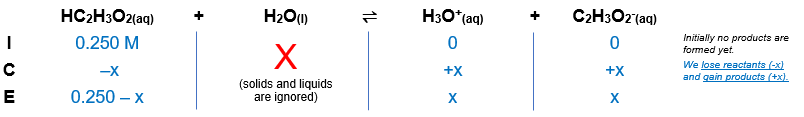# Problem: Consider the following reaction:HC2H3O2(aq) + H2O(l) ⇌ H3O+(aq) + C2H3O−2(aq)Kc = 1.8×10−5 at 25°CIf a solution initially contains 0.250 M HC2H3O2, what is the equilibrium concentration of H3O+ at 25°C?

###### FREE Expert Solution

Given:

Kc = 1.8×10−5 at 25°C

[HC2H3O2] = 0.250 M

Balanced chemical equation: HC2H3O2(aq) + H2O(l) ⇌ H3O+(aq) + C2H3O2(aq)

Step 1: Create an ICE chart.Step 2: The Kc expression for the equilibrium reaction is:

$\overline{){{\mathbf{K}}}_{{\mathbf{c}}}{\mathbf{=}}\frac{\mathbf{products}}{\mathbf{reactants}}}$

ICE table into the Kc expression:

84% (138 ratings)###### Problem Details

Consider the following reaction:

HC2H3O2(aq) + H2O(l) ⇌ H3O+(aq) + C2H3O−2(aq)

Kc = 1.8×10−5 at 25°C

If a solution initially contains 0.250 M HC2H3O2, what is the equilibrium concentration of H3O+ at 25°C?